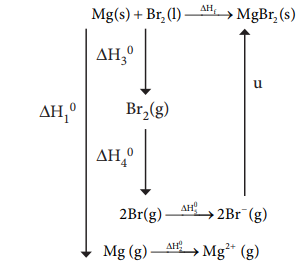Home | | Chemistry 11th std | Evaluate Yourself: Thermodynamics(Chemistry)

# Evaluate Yourself: Thermodynamics(Chemistry)

Chemistry : Thermodynamics : Evaluate Yourself

Evaluate Yourself

1. Calculate ŌłåHf┬░ for the reaction

CO2(g)+ H2(g) ŌåÆ CO(g)+ H2O(g)

given that ŌłåHf┬░ for CO2 (g), CO (g) and H2O(g) are ŌĆō 393.5, ŌĆō 111.31 and ŌĆō 242 kJ molŌĆō1 respectively.

Solution :

Given

ŌłåHf0 CO2 = -393.5 kJ mol-1

ŌłåHf0 CO = -111.31 kJ mol-1

ŌłåHf0 (H2O) = -242 kJ mol-1

CO2(g) + H2(g) ŌåÆ CO(g) + H2O(g)

ŌłåHr0 = ?

ŌłåHr0 = ╬Ż (ŌłåHf0 )productsŌĆō ╬Ż (ŌłåHf0)reactants

ŌłåHr0 = [ŌłåHf0 (CO) + ŌłåHf0 (H2O)] ŌĆō [ŌłåHf0 (CO2 )+ŌłåHf0

(H2)] ŌłåHr0 = [ŌĆō 111.31 + (ŌĆō 242)] ŌĆō [ŌĆō 393.5 + (0)]

ŌłåHr0 = [ŌĆō 353.31] + 393.5

ŌłåHr0 = 40.19

ŌłåHr0 = + 40.19 kJ molŌĆō1

2. Calculate the amount of heat necessary to raise 180 g of water from 25┬░ C to 100┬░ C. Molar heat capacity of water is 75.3 J mol-1 K-1

Solution :

Given :

number of moles of water n

=180g / (18g mol-1  )= 10 mol

molar heat capacity of water

CP = 75.3 J KŌĆō1 molŌĆō1

T2 = 1000 C = 373 K

T1 = 250 C= 298 K

╬öH = nCP (T2 ŌĆō T1)

╬öH = 10 mol ├Ś 75.3 J molŌĆō1 KŌĆō1 ├Ś (373 ŌĆō 298) K

╬öH = 56475 J

╬öH = 56.475 kJ

3. From the following data at constant volume for combustion of benzene, calculate the heat of this reaction at constant pressure condition.

C6H6(l) + 7┬Į O2(g) ŌåÆ 6CO2 (g) + 3 H2O(l)

╬öU at 25┬░ C = -3268.12 kJ

Solution :

T = 250 C = 298 K ;

ŌłåU = ŌĆō3268.12 kJ molŌĆō1

ŌłåH = ?

ŌłåH = ŌłåU + ŌłångRT

ŌłåH = ŌłåU + (np ŌĆō nr) RT

H = -3268.12+ (6 - 7/2) x 8 314 ├Ś 10  -3 x 298.

ŌłåU = ŌĆō3268.12  + (2.5 ├Ś 8.314 ├Ś10-3 ├Ś 298)

ŌłåU = ŌĆō 3268.12 + 6.19

ŌłåU = ŌĆō 3261.93 kJ molŌĆō1

4. When a mole of magnesium bromide is prepared from 1 mole of magnesium

and 1 mole of liquid bromine, 524 kJ of energy is released.

The heat of sublimation of Mg metal is 148 kJ molŌĆō1. The heat of dissociation of bromine gas into atoms is 193 kJ molŌĆō1. The heat of vapourisation of liquid bromine is 31 kJ molŌĆō1. The ionisation energy of magnesium is 2187 kJ molŌĆō1 and the electron affinity of bromine is ŌĆō 662 kJ molŌĆō1. Calculate the lattice energy of magnesium bromide.

Solution :

Given :

Mg(S) + Br2(1) ŌåÆ

MgBr2(S) ŌłåHf0 = ŌĆō524 KJ molŌĆō1

Sublimation :

Mg(S) ŌåÆ Mg(g)

ŌłåH10 = +148 KJ molŌĆō1

Ionisation:

Mg(g) ŌåÆ Mg2+(g) + 2eŌĆō

ŌłåH20 = 2187 KJ molŌĆō1

Br2(1) ŌåÆ Br2(g)

ŌłåH30 = +31 KJ molŌĆō1

Dissociation :

Br2(g) ŌåÆ 2Br(g)

ŌłåH40 = +193 KJ molŌĆō1

Electron affinity:

Br(g) + e ŌĆō ŌåÆ Br ŌĆō (g)

ŌłåH50 = ŌĆō 331 KJ molŌĆō1

Solution :╬öHf  =╬öH1 + ╬öH2 + ╬öH3 + ╬öH4 +2╬öH5 + u

ŌĆō 524 =   148 + 2187 + 31 + 193+(2 ├Ś ŌĆō 331) + u

ŌĆō 524 =   1897 + u

u =ŌĆō524 ŌĆō 1897

u =ŌĆō 2421 kJ molŌĆō1

5. An engine operating between 1270 C and 470 C takes some specified amount of heat from a high temperature reservoir. Assuming that there are no frictional losses, calculate the percentage efficiency of an engine.

Solution:

Given

Th = 1270 C = 127 + 273 = 400

K TC = 470 C = 47 + 273 = 320

K % efficiency ╬Ę = ?6. Urea on hydrolysis produces ammonia and carbon dioxide. The standard entropies of urea, H2O, CO2, NH3 are 173.8, 70, 213.5 and 192.5J mole-1K-1 respectively. Calculate the entropy change for this reaction.

Solution:7. Calculate the entropy change when 1 mole of ethanol is evaporated at 351 K. The molar heat of vaporisation of ethanol is 39.84 kJ mol-1

Solution:8. For a chemical reaction the values of ╬öH and ╬öS at 300K are ŌłÆ 10 kJ moleŌłÆ1 and ŌłÆ20 J degŌłÆ1 moleŌłÆ1 respectively. What is the value of ╬öG of the reaction? Calculate the ╬öG of a reaction at 600 K assuming ╬öH and ╬öS values are constant. Predict the nature of the reaction.

Solution:

Given:

╬öH = ŌĆō10 kJ molŌĆō1 = ŌĆō10000 J molŌĆō1

╬öS = ŌĆō 20 J KŌĆō1 molŌĆō1

T =300 K

╬öG =?

╬öG =╬öH ŌĆō T╬öS

╬öG = ŌĆō 10 kJ molŌĆō1 ŌĆō 300 K ├Ś (ŌĆō20x10-3) kJ KŌĆō1 molŌĆō1

╬öG =(ŌĆō10+6) kJ molŌĆō1

╬öG =ŌĆō 4 kJ molŌĆō1

At 600 K

╬öG =ŌĆō 10 kJ molŌĆō1 ŌĆō 600 K

(ŌĆō20x10-3) kJ KŌĆō1 molŌĆō1

╬öG = (ŌĆō10 + 12) kJ molŌĆō1

╬öG = + 2 kJ molŌĆō1

The value of ŌłåG is negative at 300K and the reaction is spontaneous, but at 600K the value ŌłåG becomes positive and the reaction is non spontaneous.

Tags : Solved Example Problems with Answer , 11th Chemistry : UNIT 7 : Thermodynamics
Study Material, Lecturing Notes, Assignment, Reference, Wiki description explanation, brief detail
11th Chemistry : UNIT 7 : Thermodynamics : Evaluate Yourself: Thermodynamics(Chemistry) | Solved Example Problems with Answer